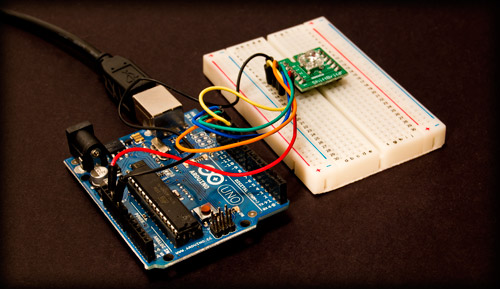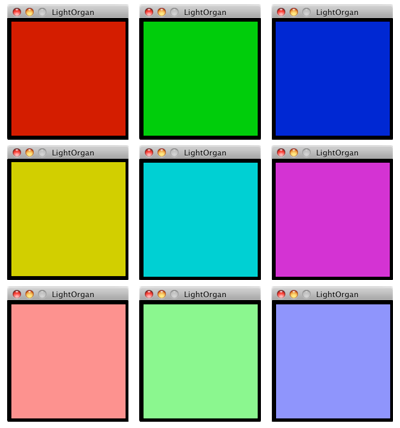Categories

## Arduino + ShiftBrite Light OrganIf you liked the last Arduino + ShiftBrite post, but don’t like Perl, here’s a Processing sketch for you… It’s a simple Light Organ that lights up the ShiftBrite in a specific color based on pressing a key.

(Once again we’re using the HughesyShiftBrite library.)

Here’s the code for the Arduino, which is pretty similar to our code from last time:

```/*
* LightOrgan.pde - Arduino
*/

#include "HughesyShiftBrite.h";

HughesyShiftBrite sb;

void setup()
sb = HughesyShiftBrite(10,11,12,13);
sb.sendColour(0,0,0);
Serial.begin(9600);
}

void loop() {

switch (input)  {
case 48:
sb.sendColour(0,0,0);
break;
case 49:
sb.sendColour(700,0,0);
break;
case 50:
sb.sendColour(0,700,0);
break;
case 51:
sb.sendColour(0,0,700);
break;
case 52:
sb.sendColour(700,700,0);
break;
case 53:
sb.sendColour(0,700,700);
break;
case 54:
sb.sendColour(700,0,700);
break;
case 55:
sb.sendColour(900,300,300);
break;
case 56:
sb.sendColour(300,900,300);
break;
case 57:
sb.sendColour(300,300,900);
break;
}
delay(5);
}
```

The only real difference here is the colors we are using…

Once that’s loaded on the Arduino, create a new sketch in Processing with the following code:

```/*
* LightOrganController.pde - Processing
*/

import processing.serial.*;
Serial myPort;

void setup() {
println(Serial.list());
myPort = new Serial(this, Serial.list(), 9600);
myPort.buffer(1);
size(200, 200);
background(0,0,0);
}

void draw() {
// nothing needed here
}

void keyPressed() {
if (int(key) == 48) {
myPort.write(48);
fill(0,0,0);
rect(5,5,190,190);
}
else if (int(key) == 49) {
myPort.write(49);
fill(200,0,0);
rect(5,5,190,190);
}
else if (int(key) == 50) {
myPort.write(50);
fill(0,200,0);
rect(5,5,190,190);
}
else if (int(key) == 51) {
myPort.write(51);
fill(0,0,200);
rect(5,5,190,190);
}
else if (int(key) == 52) {
myPort.write(52);
fill(200,200,0);
rect(5,5,190,190);
}
else if (int(key) == 53) {
myPort.write(53);
fill(0,200,200);
rect(5,5,190,190);
}
else if (int(key) == 54) {
myPort.write(54);
fill(200,0,200);
rect(5,5,190,190);
}
else if (int(key) == 55) {
myPort.write(55);
fill(250,125,125);
rect(5,5,190,190);
}
else if (int(key) == 56) {
myPort.write(56);
fill(125,250,125);
rect(5,5,190,190);
}
else if (int(key) == 57) {
myPort.write(57);
fill(125,125,250);
rect(5,5,190,190);
}
else {
myPort.write(48);
fill(0,0,0);
rect(5,5,190,190);
}
}
```

When you run the Processing code, it will draw a black box on the screen, and if you press any number between 0 and 9 it will change the color of the box, as well as changing the color of the ShiftBrite.If you’re lucky enough to have a number keypad on your keyboard (I mean, since Apple stopped including that part of the keyboard) you can go nuts hitting all the keys from 1 through 9 (and zero for “off” or black) and put on a nice little light show.

If you don’t care about the on screen display and just want to control the ShiftBrite, here’s some simpler code:

```import processing.serial.*;
Serial myPort;

void setup() {
println(Serial.list());
myPort = new Serial(this, Serial.list(), 9600);
myPort.buffer(1);
}

void draw() {
// nothing needed here
}

void keyPressed() {
if ((int(key) > 47) & (int(key) < 58)) {
myPort.write(int(key));
}
else {
myPort.write(48);
}
}
```

Since we don’t need to draw anything based on the key pressed, we just look for a key between 0 and 9 and send it over the serial port.

Disclaimer: This code is probably pretty damn basic, but I’m a fan of documenting the process, and I’m still learning (and having fun!) and I hope someone finds it useful. If you’ve got suggestions or improvements, let me know… thanks!

Categories

## Accelerometer Art

At MilwaukeeDevHouse5 Matt and I played with Arduinos, so here, with the fairly uninspiring name of “Accelerometer Art” I present three screen shots of a Processing application displaying data from an ADXL335 accelerometer connected to my MacBook via an Arduino.

Here’s the code that runs on the Arduino…

```/*
* Accelerometer.pde
*/

#define aref_voltage 3.3

int xpin = 1;
int ypin = 2;
int zpin = 3;

void setup(void) {
Serial.begin(9600);
analogReference(EXTERNAL);
}

void loop(void) {

Serial.print (xval);
Serial.print (" ");
Serial.print (yval);
Serial.print (" ");
Serial.print (zval);
Serial.print (" \n");

delay(10);
}
```

And here’s the code that runs in Processing…

```/*
* Accelerometer_Graph.pde
*/

import processing.serial.*;

Serial myPort;
int xPos = 0;
int yPos = 0;
int zPos = 0;

void setup () {
size(1024, 768);
println(Serial.list());
myPort = new Serial(this, Serial.list(), 9600);
myPort.bufferUntil('\n');
background(0);
}

void draw () {
// everything happens in the serialEvent()
}

void serialEvent (Serial myPort) {

String[] nums = split(inString, ' ');
String inStringx = nums;
String inStringy = nums;
String inStringz = nums;

if (inStringx != null) {
inStringx = trim(inStringx);
float inBytex = float(inStringx);
inBytex = map(inBytex, 0, 1023, 0, height);

stroke(255,0,0);
point(xPos, height - inBytex);
strokeWeight(3);

if (xPos >= width) {
xPos = 1;
background(0);
}
else {
xPos = xPos + 1;
}
}

if (inStringy != null) {
inStringy = trim(inStringy);
float inBytey = float(inStringy);
inBytey = map(inBytey, 0, 1023, 0, height);

stroke(0,255,0);
point(yPos, height - inBytey);
strokeWeight(3);

if (yPos >= width) {
yPos = 2;
background(0);
}
else {
yPos = yPos + 1;
}
}

if (inStringz != null) {
inStringz = trim(inStringz);
float inBytez = float(inStringz);
inBytez = map(inBytez, 0, 1023, 0, height);

stroke(0,0,255);
point(zPos, height - inBytez);
strokeWeight(3);

if (zPos >= width) {
zPos = 3;
background(0);
}
else {
zPos = zPos + 1;
}
}
}

void keyPressed() {
if (int(key) == 113) {
exit();
}
}
```

The Processing code was based on an example from Tom Igoe which he placed in the public domain. (Thanks Tom!)

I heavily violated the DRY rule with this code, so it should really be re-written to be more efficient. Besides all that, it does actually work, as you can see from the awesome graphics above. (Thanks to Matt Gauger for helping with the code, and yes, he scolded me for violating the DRY rule.)

This was a great first step into Processing for me, and I look forward to improving this code, as well as explore some other ideas I have for graphing data.

(If you’ve got any pointers to great tutorials, blogs, or web sites focusing on Processing, let me know!)